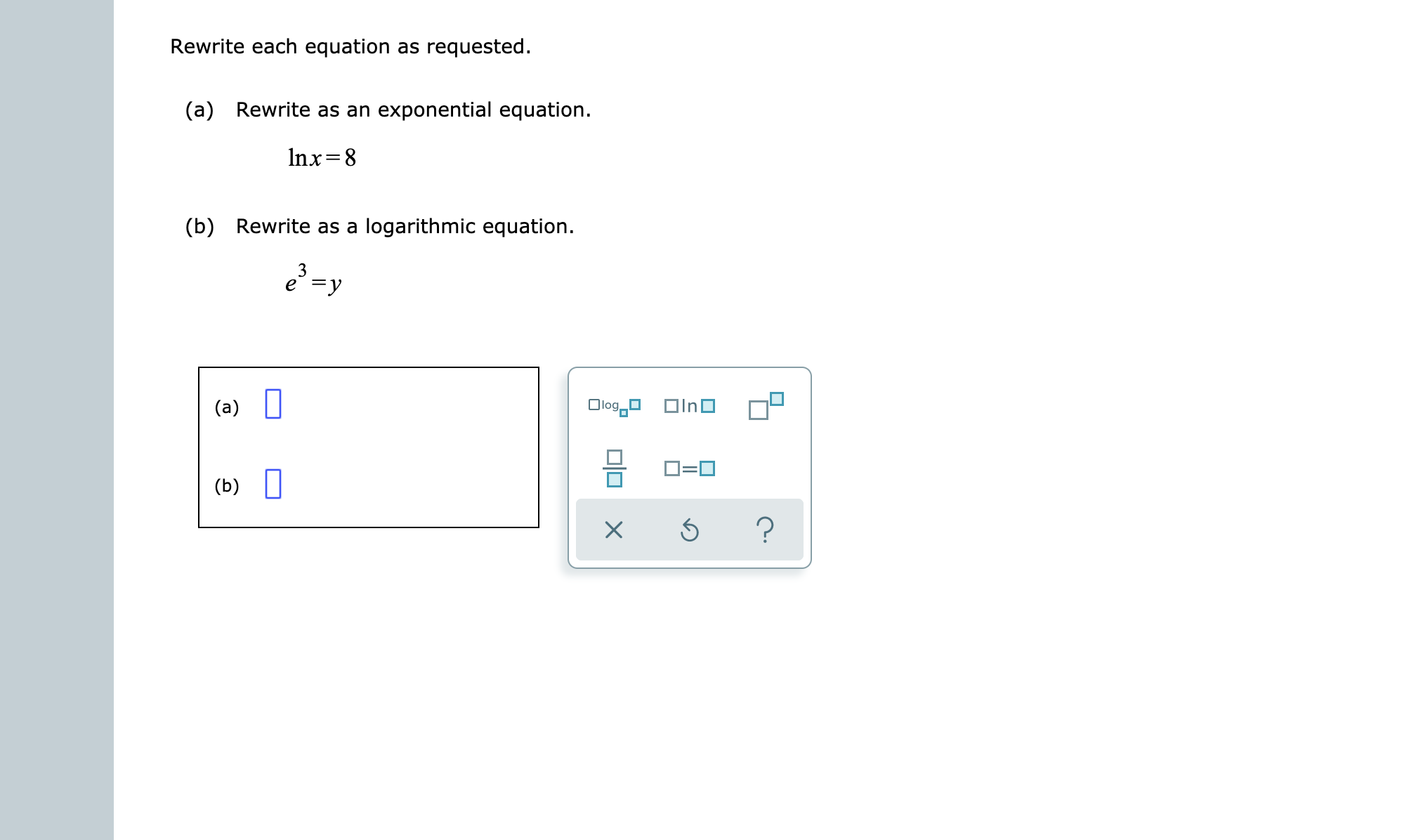# Rewrite each equation as requested.Rewrite as an exponential equation.(a)Inx 8Rewrite as a logarithmic equation(b)3e yOlogInD(a)(b)

Question
7 views

see attachmenthelp_outlineImage TranscriptioncloseRewrite each equation as requested. Rewrite as an exponential equation. (a) Inx 8 Rewrite as a logarithmic equation (b) 3 e y Olog InD (a) (b) fullscreen
check_circle

Step 1

Part(a):

Given:

Step 2

Taking exponential both side of the given function:

Step 3

Part(b):

Give...

### Want to see the full answer?

See Solution

#### Want to see this answer and more?

Solutions are written by subject experts who are available 24/7. Questions are typically answered within 1 hour.*

See Solution
*Response times may vary by subject and question.
Tagged in

### Calculus